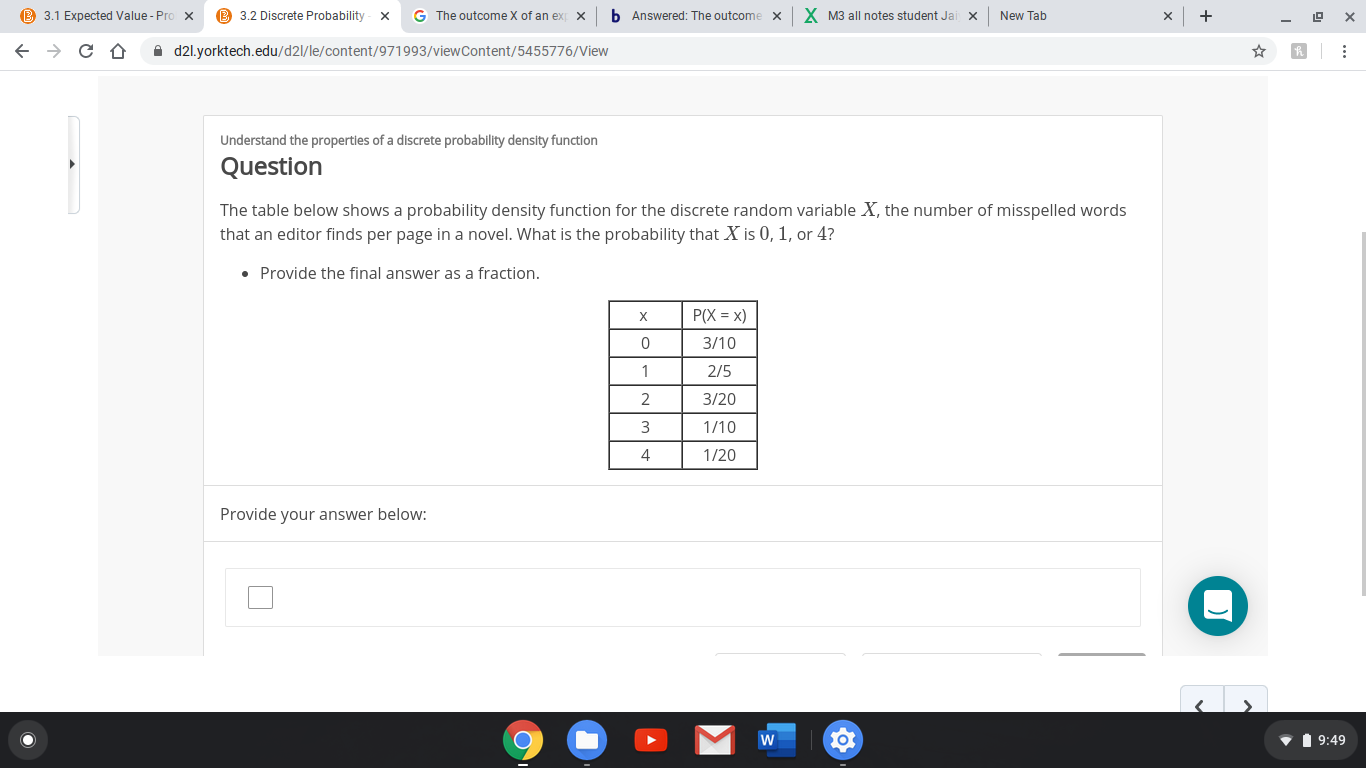# B 3.1 Expected Value - Pro xB 3.2 Discrete Probabilityb Answered: The outcomeX M3 all notes student JaiG The outcome X of an exNew TabxxA d2l.yorktech.edu/d2l/le/content/971993/viewContent/5455776/ViewUnderstand the properties of a discrete probability density functionQuestionThe table below shows a probability density function for the discrete random variable X, the number of misspelled wordsthat an editor finds per page in a novel. What is the probability that X is 0, 1, or 4?• Provide the final answer as a fraction.P(X = x)3/102/53/201/101/20Provide your answer below:V 1 9:49

Question
1 views

The table below shows a probability density function for the discrete random variable X, the number of misspelled words that an editor finds per page in a novel. What is the probability that X is 0, 1, or 4?

• Provide the final answer as a fraction.help_outlineImage TranscriptioncloseB 3.1 Expected Value - Pro x B 3.2 Discrete Probability b Answered: The outcome X M3 all notes student Jai G The outcome X of an ex New Tab x x A d2l.yorktech.edu/d2l/le/content/971993/viewContent/5455776/View Understand the properties of a discrete probability density function Question The table below shows a probability density function for the discrete random variable X, the number of misspelled words that an editor finds per page in a novel. What is the probability that X is 0, 1, or 4? • Provide the final answer as a fraction. P(X = x) 3/10 2/5 3/20 1/10 1/20 Provide your answer below: V 1 9:49 fullscreen
check_circle

Step 1

It is given that X denotes ...

### Want to see the full answer?

See Solution

#### Want to see this answer and more?

Solutions are written by subject experts who are available 24/7. Questions are typically answered within 1 hour.*

See Solution
*Response times may vary by subject and question.
Tagged in

### Basic Probability